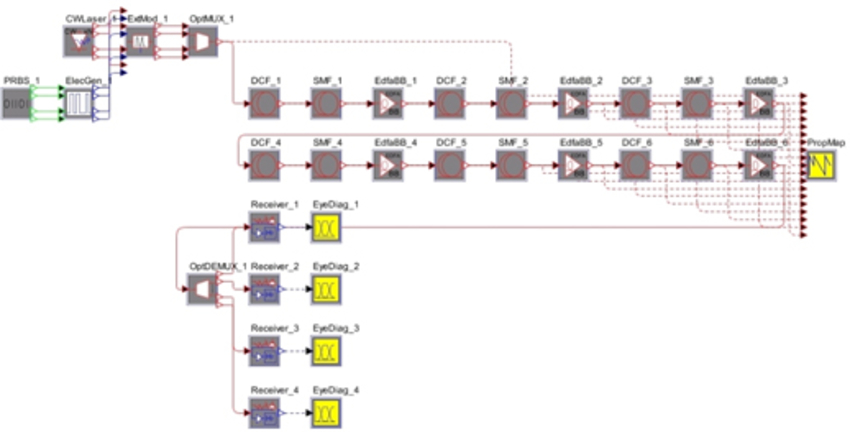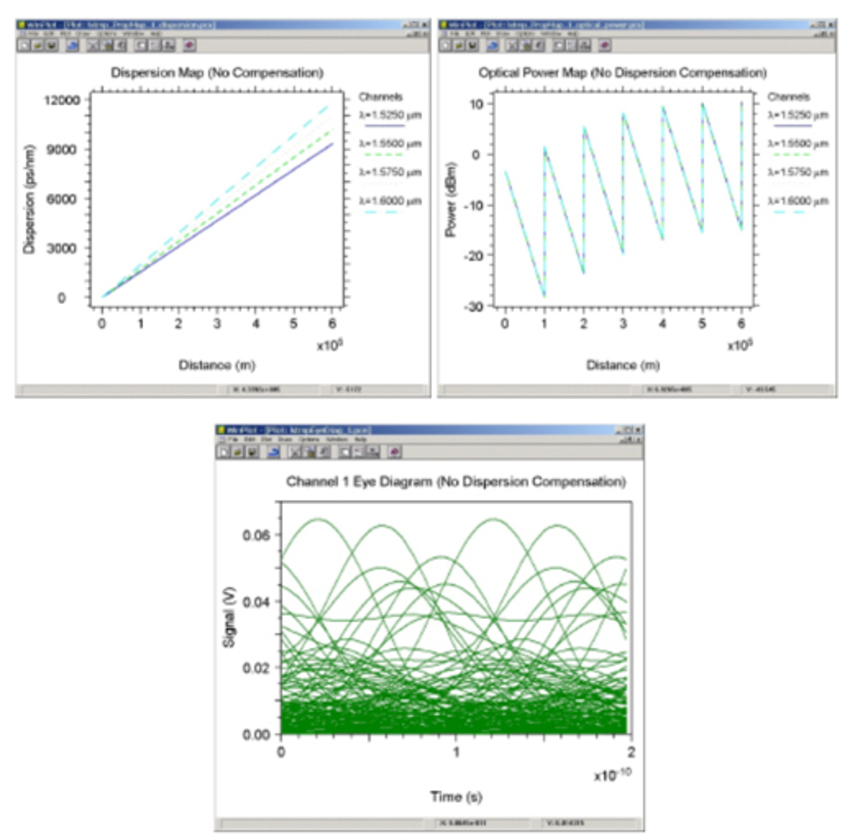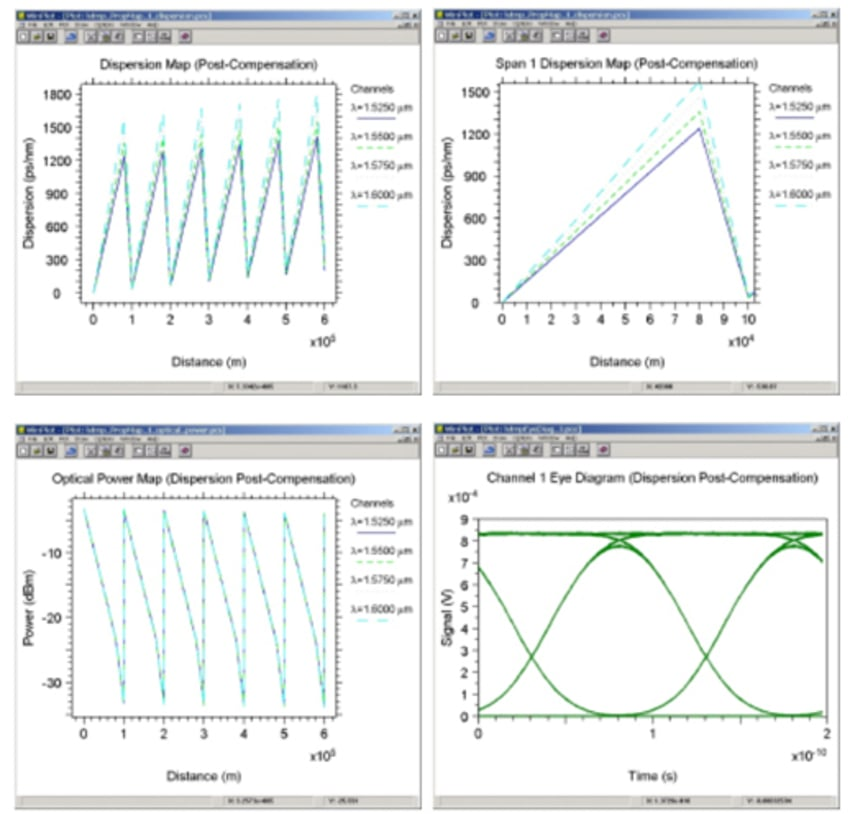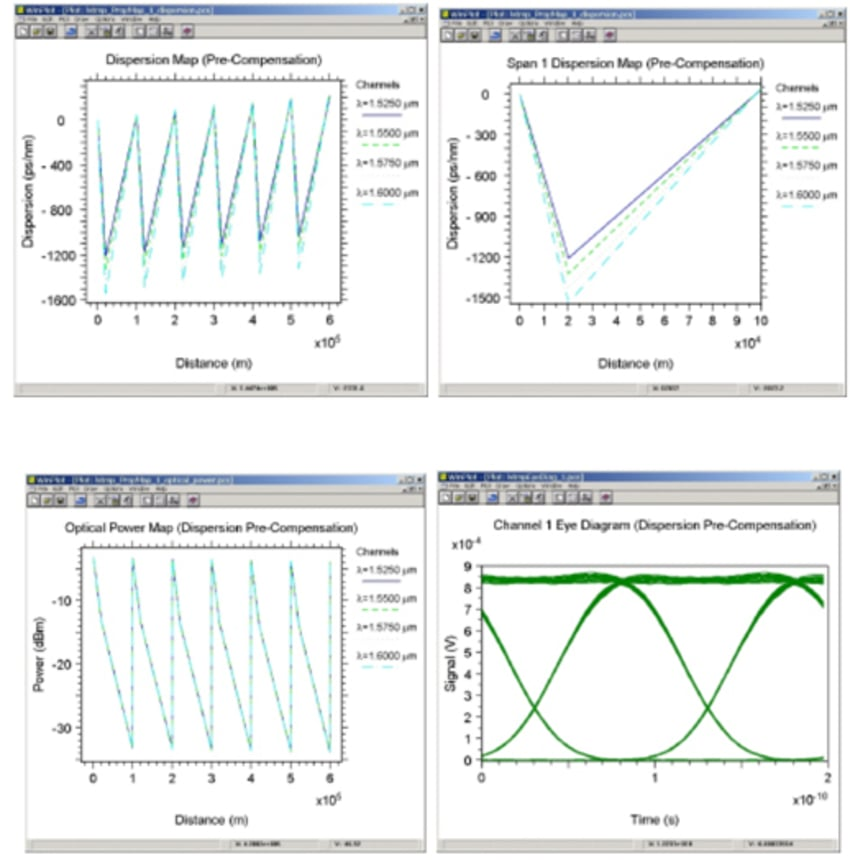# Electronic Dispersion Compensation

## Tools Used: OptSim

Different spectral components of the launched optical pulse travel within the fiber at slightly different group velocities. This spreads the pulse in the time domain producing the effect known as chromatic dispersion (CD). The following example illustrates the use of OptSim in studying dispersion compensation in a long-haul high-speed WDM system, and demonstrates how OptSim can help in understanding, evaluating and optimizing the chromatic dispersion compensation methods.

Dispersion management in single mode fiber links can be accomplished in many ways, though the most widely used approach employs lengths of transport fiber of opposite dispersion characteristics to the principal fiber in the link, usually standard singlemode fiber (SSMF). Typically a 10 to 20km length of dispersion compensating fiber (DCF) is placed before the regenerators inducing negative dispersion to compensate for the positive dispersion accumulated over the 60 to 80km length of the SMF. Although the total dispersion over the entire SMF-DCF span can be minimal, net dispersion at any point along the span is non-zero, which keeps the nonlinear mixing effects at minimum levels.

The figure below demonstrates the layout that has a total link-length of 600 km. Each 100-km span consists of 80 km of ITU-T G.652 SMF and 20 km of DCF. The total data rate of the 4-channel WDM link is 40 Gbps. The wavelength plan is a non-ITU-T grid with widely placed channels in order to illustrate clearly the distinct dispersion slopes for each wavelength.The following figure shows performance for an uncompensated (i.e., SMF span = 100km, and DCF span = 0km) link. The power map rises with the link length because the amplifier over-compensates for the attenuation at each span. Since there is no dispersion scheme employed, the accumulated dispersion for each wavelength produces a closed eye at the receiver even if the link is not power-limited.On the other hand, if we use DCF with a high figure of merit (FOM) to compensate for the accumulated dispersion, we can see On the other hand, if we use DCF with a high figure of merit (FOM) to compensate for the accumulated dispersion, we can see (see eye diagram on the bottom right) an impressive improvement in the performance. The upper left diagram shows the dispersion maps for all four channels. The unequal accumulated dispersion at the end of each SMF segment for each wavelength is due to the wavelength-dependent dispersion in the fiber, also known as the dispersion slope. The power map in the bottom left diagram shows that the power falls more rapidly in the DCF section than in the SMF section because of the larger attenuation typically associated with the DCF. A closer look at the Span 1 dispersion map (Figure 3(b)) shows that the net dispersion at the end of the span is non-zero, but minimal, and does not materially affect the simulation results in this example.an impressive improvement in the performance. Figure 3(a) shows the dispersion maps for all four channels. The unequal accumulated dispersion at the end of each SMF segment for each wavelength is due to the wavelength-dependent dispersion in the fiber, also known as the dispersion slope. The power map in Figure 3(c) shows that the power falls more rapidly in the DCF section than in the SMF section because of the larger attenuation typically associated with the DCF. A closer look at the Span 1 dispersion map (upper right) shows that the net dispersion at the end of the span is non-zero, but minimal, and does not materially affect the simulation results in this example.For the same layout, if we interchange the placements of DCF and SMF (i.e., DCF followed by SMF), the corresponding set of diagrams is shown in the following figure:A careful comparison of the eye diagrams reveals that the pre-compensation scheme produces a slight improvement in performance over the post-compensation scheme. This is because pre-compensation decreases the signal power faster than post-compensation due to the higher attenuation of the DCF, and the signal experiences normal dispersion while the signal power is higher; whereas in post-compensation, the signal power falls more slowly in the SMF and experiences anomalous dispersion while the signal power is higher. Pre-compensation has also been shown to result in pulse compression due to self-phase modulation (SPM), rather than the more detrimental pulse broadening effect that occurs in post-compensation. Due to the interplay between dispersion, nonlinearities, and signal power, the dispersion map strongly affects the pulse evolution in the link.